# Geometry quiz

## Related software (5)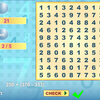## Math Search

Solve the equations and look for the answers on the grid.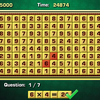## Arithmetic Game

Complete the equations in the interesting arithmetic test!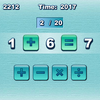## Quick Math

How quickly can you complete the equations with arithmetic symbols?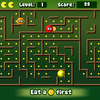## Math Man

Play Ghost Man with a mathematical twist!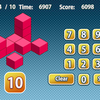## Count the Cubes

Can you spot the exact number of cubes immediately?